# 7.1 Greatest common factor and factor by grouping  (Page 3/5)

 Page 3 / 5

Factor: $-16z-64$ .

$-8\left(8z+8\right)$

Factor: $-9y-27$ .

$-9\left(y+3\right)$

Factor: $-6{a}^{2}+36a$ .

## Solution

The leading coefficient is negative, so the GCF will be negative.?

 Since the leading coefficient is negative, the GCF is negative, −6 a .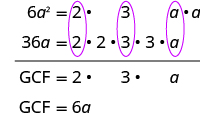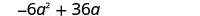Rewrite each term using the GCF.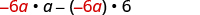Factor the GCF.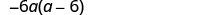Check. $-6a\left(a-6\right)$ $-6a\cdot a+\left(-6a\right)\left(-6\right)$ $-6{a}^{2}+36a✓$

Factor: $-4{b}^{2}+16b$ .

$-4b\left(b-4\right)$

Factor: $-7{a}^{2}+21a$ .

$-7a\left(a-3\right)$

Factor: $5q\left(q+7\right)-6\left(q+7\right)$ .

## Solution

The GCF is the binomial $q+7$ .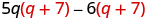Factor the GCF, ( q + 7).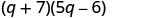Check on your own by multiplying.

Factor: $4m\left(m+3\right)-7\left(m+3\right)$ .

$\left(m+3\right)\left(4m-7\right)$

Factor: $8n\left(n-4\right)+5\left(n-4\right)$ .

$\left(n-4\right)\left(8n+5\right)$

## Factor by grouping

When there is no common factor of all the terms of a polynomial, look for a common factor in just some of the terms. When there are four terms, a good way to start is by separating the polynomial into two parts with two terms in each part. Then look for the GCF in each part. If the polynomial can be factored, you will find a common factor emerges from both parts.

(Not all polynomials can be factored. Just like some numbers are prime, some polynomials are prime.)

## How to factor by grouping

Factor: $xy+3y+2x+6$ .

## Solution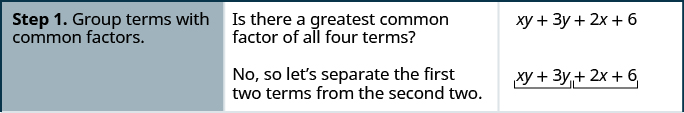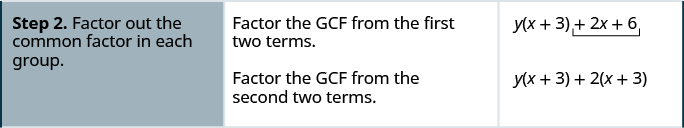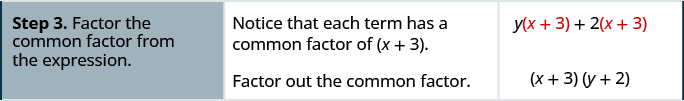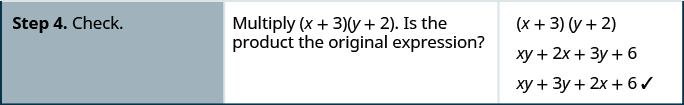Factor: $xy+8y+3x+24$ .

$\left(x+8\right)\left(y+3\right)$

Factor: $ab+7b+8a+56$ .

$\left(a+7\right)\left(b+8\right)$

## Factor by grouping.

1. Group terms with common factors.
2. Factor out the common factor in each group.
3. Factor the common factor from the expression.
4. Check by multiplying the factors.

Factor: ${x}^{2}+3x-2x-6$ .

## Solution

$\begin{array}{cccc}\text{There is no GCF in all four terms.}\hfill & & & \hfill \phantom{\rule{4em}{0ex}}{x}^{2}+3x\phantom{\rule{0.5em}{0ex}}-2x-6\hfill \\ \text{Separate into two parts.}\hfill & & & \hfill \phantom{\rule{4em}{0ex}}\underset{⎵}{{x}^{2}+3x}\phantom{\rule{0.5em}{0ex}}\underset{⎵}{-2x-6}\hfill \\ \\ \\ \begin{array}{c}\text{Factor the GCF from both parts. Be careful}\hfill \\ \text{with the signs when factoring the GCF from}\hfill \\ \text{the last two terms.}\hfill \end{array}\hfill & & & \hfill \phantom{\rule{4em}{0ex}}\begin{array}{c}\hfill x\left(x+3\right)-2\left(x+3\right)\hfill \\ \hfill \left(x+3\right)\left(x-2\right)\hfill \end{array}\hfill \\ \\ \\ \text{Check on your own by multiplying.}\hfill & & & \end{array}$

Factor: ${x}^{2}+2x-5x-10$ .

$\left(x-5\right)\left(x+2\right)$

Factor: ${y}^{2}+4y-7y-28$ .

$\left(y+4\right)\left(y-7\right)$

Access these online resources for additional instruction and practice with greatest common factors (GFCs) and factoring by grouping.

## Key concepts

• Finding the Greatest Common Factor (GCF): To find the GCF of two expressions:
1. Factor each coefficient into primes. Write all variables with exponents in expanded form.
2. List all factors—matching common factors in a column. In each column, circle the common factors.
3. Bring down the common factors that all expressions share.
4. Multiply the factors as in [link] .
• Factor the Greatest Common Factor from a Polynomial: To factor a greatest common factor from a polynomial:
1. Find the GCF of all the terms of the polynomial.
2. Rewrite each term as a product using the GCF.
3. Use the ‘reverse’ Distributive Property to factor the expression.
4. Check by multiplying the factors as in [link] .
• Factor by Grouping: To factor a polynomial with 4 four or more terms
1. Group terms with common factors.
2. Factor out the common factor in each group.
3. Factor the common factor from the expression.
4. Check by multiplying the factors as in [link] .

## Practice makes perfect

Find the Greatest Common Factor of Two or More Expressions

In the following exercises, find the greatest common factor.

8, 18

2

24, 40

72, 162

18

150, 275

10 a , 50

10

5 b , 30

$3x,10{x}^{2}$

$x$

$21{b}^{2},14b$

$8{w}^{2},24{w}^{3}$

$8{w}^{2}$

$30{x}^{2},18{x}^{3}$

$10{p}^{3}q,12p{q}^{2}$

$2pq$

$8{a}^{2}{b}^{3},10a{b}^{2}$

$12{m}^{2}{n}^{3},30{m}^{5}{n}^{3}$

$6{m}^{2}{n}^{3}$

$28{x}^{2}{y}^{4},42{x}^{4}{y}^{4}$

$10{a}^{3},12{a}^{2},14a$

$2a$

$20{y}^{3},28{y}^{2},40y$

$35{x}^{3},10{x}^{4},5{x}^{5}$

$5{x}^{3}$

$27{p}^{2},45{p}^{3},9{p}^{4}$

Factor the Greatest Common Factor from a Polynomial

In the following exercises, factor the greatest common factor from each polynomial.

$4x+20$

$4\left(x+5\right)$

$8y+16$

$6m+9$

$3\left(2m+3\right)$

$14p+35$

$9q+9$

$9\left(q+1\right)$

$7r+7$

$8m-8$

$8\left(m-1\right)$

$4n-4$

$9n-63$

$9\left(n-7\right)$

$45b-18$

$3{x}^{2}+6x-9$

$3\left({x}^{2}+2x-3\right)$

$4{y}^{2}+8y-4$

$8{p}^{2}+4p+2$

$2\left(4{p}^{2}+2p+1\right)$

$10{q}^{2}+14q+20$

$8{y}^{3}+16{y}^{2}$

$8{y}^{2}\left(y+2\right)$

$12{x}^{3}-10x$

$5{x}^{3}-15{x}^{2}+20x$

$5x\left({x}^{2}-3x+4\right)$

$8{m}^{2}-40m+16$

$12x{y}^{2}+18{x}^{2}{y}^{2}-30{y}^{3}$

$6{y}^{2}\left(2x+3{x}^{2}-5y\right)$

$21p{q}^{2}+35{p}^{2}{q}^{2}-28{q}^{3}$

$-2x-4$

$-2\left(x+4\right)$

$-3b+12$

$5x\left(x+1\right)+3\left(x+1\right)$

$\left(x+1\right)\left(5x+3\right)$

$2x\left(x-1\right)+9\left(x-1\right)$

$3b\left(b-2\right)-13\left(b-2\right)$

$\left(b-2\right)\left(3b-13\right)$

$6m\left(m-5\right)-7\left(m-5\right)$

Factor by Grouping

In the following exercises, factor by grouping.

$xy+2y+3x+6$

$\left(y+3\right)\left(x+2\right)$

$mn+4n+6m+24$

$uv-9u+2v-18$

$\left(u+2\right)\left(v-9\right)$

$pq-10p+8q-80$

${b}^{2}+5b-4b-20$

$\left(b-4\right)\left(b+5\right)$

${m}^{2}+6m-12m-72$

${p}^{2}+4p-9p-36$

$\left(p-9\right)\left(p+4\right)$

${x}^{2}+5x-3x-15$

Mixed Practice

In the following exercises, factor.

$-20x-10$

$-10\left(2x+1\right)$

$5{x}^{3}-{x}^{2}+x$

$3{x}^{3}-7{x}^{2}+6x-14$

$\left({x}^{2}+2\right)\left(3x-7\right)$

${x}^{3}+{x}^{2}-x-1$

${x}^{2}+xy+5x+5y$

$\left(x+y\right)\left(x+5\right)$

$5{x}^{3}-3{x}^{2}-5x-3$

## Everyday math

Area of a rectangle The area of a rectangle with length 6 less than the width is given by the expression ${w}^{2}-6w$ , where $w=$ width. Factor the greatest common factor from the polynomial.

$w\left(w-6\right)$

Height of a baseball The height of a baseball t seconds after it is hit is given by the expression $-16{t}^{2}+80t+4$ . Factor the greatest common factor from the polynomial.

## Writing exercises

The greatest common factor of 36 and 60 is 12. Explain what this means.

Answers will vary.

What is the GCF of ${y}^{4},{y}^{5},\text{and}\phantom{\rule{0.2em}{0ex}}{y}^{10}$ ? Write a general rule that tells you how to find the GCF of ${y}^{a},{y}^{b},\text{and}{y}^{c}$ .

## Self check

After completing the exercises, use this checklist to evaluate your mastery of the objectives of this section.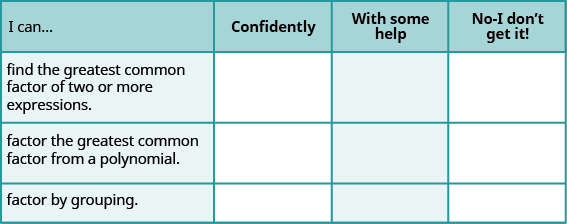If most of your checks were:

…confidently. Congratulations! You have achieved your goals in this section! Reflect on the study skills you used so that you can continue to use them. What did you do to become confident of your ability to do these things? Be specific!

…with some help. This must be addressed quickly as topics you do not master become potholes in your road to success. Math is sequential—every topic builds upon previous work. It is important to make sure you have a strong foundation before you move on. Who can you ask for help? Your fellow classmates and instructor are good resources. Is there a place on campus where math tutors are available? Can your study skills be improved?

…no - I don’t get it! This is critical and you must not ignore it. You need to get help immediately or you will quickly be overwhelmed. See your instructor as soon as possible to discuss your situation. Together you can come up with a plan to get you the help you need.

#### Questions & Answers

find the solution to the following functions, check your solutions by substitution. f(x)=x^2-17x+72
Carlos Reply
Aziza is solving this equation-2(1+x)=4x+10
Sechabe Reply
No. 3^32 -1 has exactly two divisors greater than 75 and less than 85 what is their product?
KAJAL Reply
x^2+7x-19=0 has Two solutions A and B give your answer to 3 decimal places
Adedamola Reply
please the answer to the example exercise
Patricia Reply
3. When Jenna spent 10 minutes on the elliptical trainer and then did circuit training for20 minutes, her fitness app says she burned 278 calories. When she spent 20 minutes onthe elliptical trainer and 30 minutes circuit training she burned 473 calories. How manycalories does she burn for each minute on the elliptical trainer? How many calories doesshe burn for each minute of circuit training?
Edwin Reply
.473
Angelita
?
Angelita
John left his house in Irvine at 8:35 am to drive to a meeting in Los Angeles, 45 miles away. He arrived at the meeting at 9:50. At 3:30 pm, he left the meeting and drove home. He arrived home at 5:18.
DaYoungan Reply
p-2/3=5/6 how do I solve it with explanation pls
Adedamola Reply
P=3/2
Vanarith
1/2p2-2/3p=5p/6
James
don't understand answer
Cindy
4.5
Ruth
is y=7/5 a solution of 5y+3=10y-4
Adedamola Reply
yes
James
don't understand answer
Cindy
Lucinda has a pocketful of dimes and quarters with a value of $6.20. The number of dimes is 18 more than 3 times the number of quarters. How many dimes and how many quarters does Lucinda have? Rhonda Reply Find an equation for the line that passes through the point P ( 0 , − 4 ) and has a slope 8/9 . Gabriel Reply is that a negative 4 or positive 4? Felix y = mx + b Felix if negative -4, then -4=8/9(0) + b Felix -4=b Felix if positive 4, then 4=b Felix then plug in y=8/9x - 4 or y=8/9x+4 Felix Macario is making 12 pounds of nut mixture with macadamia nuts and almonds. macadamia nuts cost$9 per pound and almonds cost $5.25 per pound. how many pounds of macadamia nuts and how many pounds of almonds should macario use for the mixture to cost$6.50 per pound to make?
Cherry Reply
Nga and Lauren bought a chest at a flea market for $50. They re-finished it and then added a 350 % mark - up Makaila Reply$1750
Cindy
the sum of two Numbers is 19 and their difference is 15
Abdulai Reply
2, 17
Jose
interesting
saw
4,2
Cindy
Felecia left her home to visit her daughter, driving 45mph. Her husband waited for the dog sitter to arrive and left home 20 minutes, or 13 hour later. He drove 55mph to catch up to Felecia. How long before he reaches her?
Rafi Reply
hola saben como aser un valor de la expresión
NAILEA

### Read also:

#### Get Jobilize Job Search Mobile App in your pocket Now!

Source:  OpenStax, Elementary algebra. OpenStax CNX. Jan 18, 2017 Download for free at http://cnx.org/content/col12116/1.2
Google Play and the Google Play logo are trademarks of Google Inc.

Notification Switch

Would you like to follow the 'Elementary algebra' conversation and receive update notifications?By RhodesBy OpenStaxBy Madison ChristianBy Anindyo MukhopadhyayBy OpenStaxBy IES PortalBy Cath YuBy Nick SwainBy Madison ChristianBy Jemekia Weeden